# Probability theory: Chi-Squared distribution

This is part of the course “Probability Theory and Statistics for Programmers”.

The Chi-Squared distribution is a continuous probability distribution that is widely used in statistical inference. And it related to the standard normal distribution. If a random variable X has the standard normal distribution, then has the the Chi-Squared distribution with one degree of freedom.

A relation between standard normal distribution and Chi-Squared distribution goes father than this. If we have n independent standard normal random variables, then their sum has a Chi-Squared distribution with n degrees of freedom.

Now let’s plot Chi-Squared distribution with different degrees of freedom.

The mean and variance of Chi-Squared distribution:

Next part ->

Reach the next level of focus and productivity with increaser.org.

--

--

--

Indie hacker behind increaser.org. More at radzion.com

Love podcasts or audiobooks? Learn on the go with our new app.

## Combinatorics: permutations, combinations and dispositions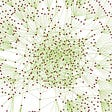## Vedic Maths: Fast Calculation Tricks## Distributions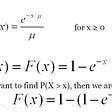## Expectation & Variance of OLS Estimates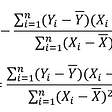## Algebra 1000\$ Meme Contest## The “Solunar” Situational Development and Outcome Spread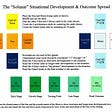## 3 Color Algorithm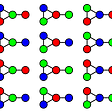## Visualizing Eigenvalues and Eigenvectors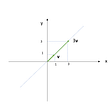Indie hacker behind increaser.org. More at radzion.com

## 2. ARMA Process — Introduction## 3 things to know to get started with time series## Linear Regression Visualized and Better Understood